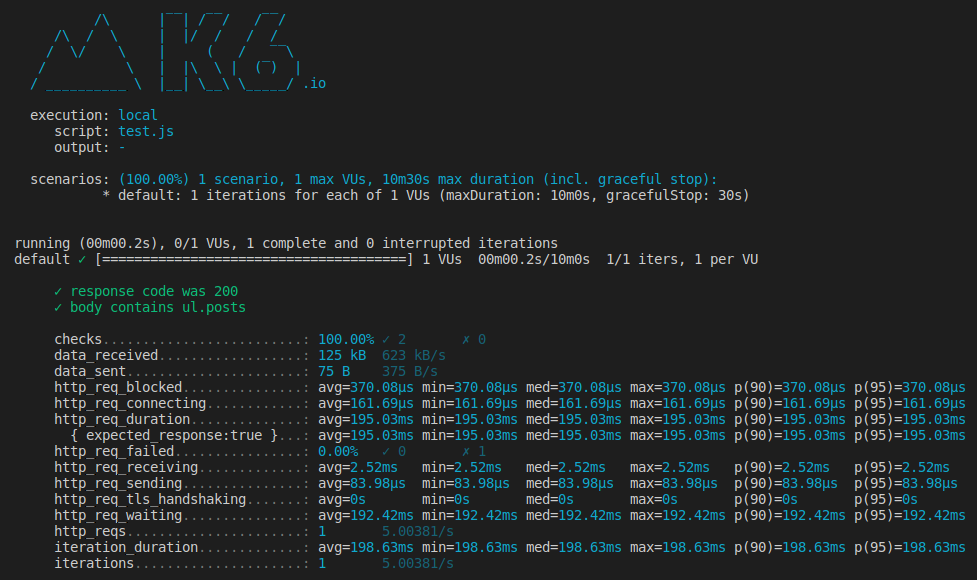# k6 check function

## What is check function in k6?

Let say you are writing a load test script where you need to perform some checks when you recieve the response for given endpoint.

You can use check function to add checks in your load test script. Following is the function signature:

check( val, sets, [tags] )

Let's write a simple script which calls localhost endpoint and does two checks. We want to perform following checks in our script:

• First if we have got 200 status
• Secondly, if we have ul element with class name posts in our response body

Let's look at following sample script:

```import { check } from "k6";
import http from "k6/http";
import { parseHTML } from "k6/html";

export default function () {
const response = http.get("http://localhost");

check(response, {
"response code was 200": (res) => res.status == 200,
"body contains ul.posts": (res) => {
const html = parseHTML(res.body);
return html.find("ul.posts").get(0).nodeName() === "ul";
},
});
}```

Now, we need to run our script on terminal window. Open your terminal and run following command:

`k6 run test.js`

Above command will give you following output: